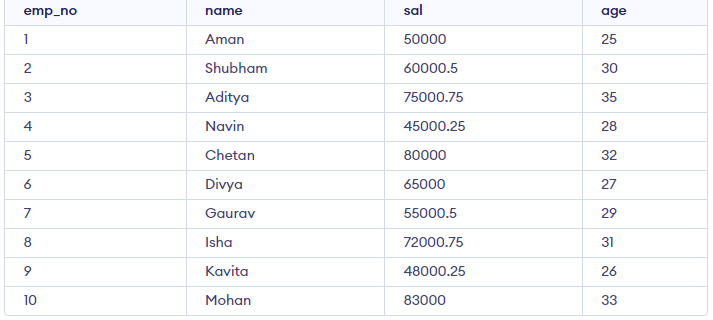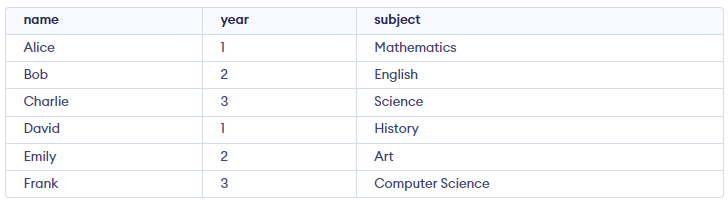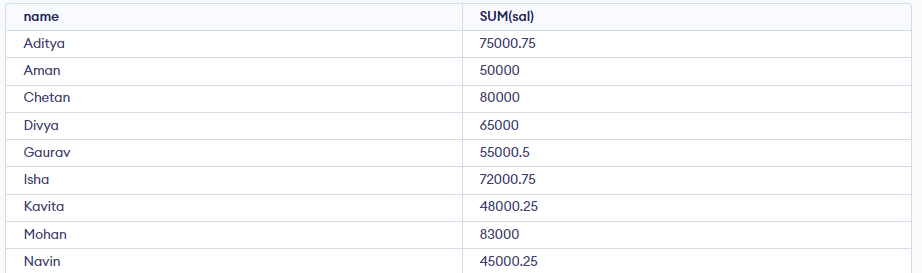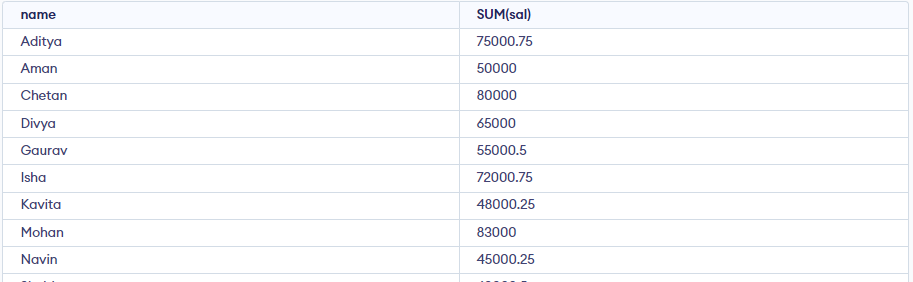# SQL | GROUP BY

The GROUP BY Statement in SQL is used to arrange identical data into groups with the help of some functions. i.e. if a particular column has the same values in different rows then it will arrange these rows in a group.

### Features

• GROUP BY clause is used with the SELECT statement.
• In the query, the GROUP BY clause is placed after the WHERE clause.
• In the query, the GROUP BY clause is placed before the ORDER BY clause if used.
• In the query, the Group BY clause is placed before the Having clause.
• Place condition in the having clause.

Syntax:

SELECT column1, function_name(column2)

FROM table_name

WHERE condition

GROUP BY column1, column2

ORDER BY column1, column2;

Explanation:

1. function_name: Name of the function used for example, SUM() , AVG().
2. table_name: Name of the table.
3. condition: Condition used.

Let’s assume that we have two tables Employee and Student Sample Table is as follows after adding two tables we will do some specific operations to learn about GROUP BY.

Employee Table:

```CREATE TABLE emp (
emp_no INT PRIMARY KEY,
name VARCHAR(50),
sal DECIMAL(10,2),
age INT
);```

Insert some random data into a table and then we will perform some operations in GROUP BY.

Query:

```INSERT INTO emp (emp_no, name, sal, age) VALUES
(1, 'Aarav', 50000.00, 25),
(3, 'Amit', 75000.75, 35),
(4, 'Anjali', 45000.25, 28),
(5, 'Chetan', 80000.00, 32),
(6, 'Divya', 65000.00, 27),
(7, 'Gaurav', 55000.50, 29),
(8, 'Isha', 72000.75, 31),
(9, 'Kavita', 48000.25, 26),
(10, 'Mohan', 83000.00, 33);```

Output:Student Table:

Query:

```CREATE TABLE student (
name VARCHAR(50),
year INT,
subject VARCHAR(50)
);
INSERT INTO student (name, year, subject) VALUES
('Alice', 1, 'Mathematics'),
('Bob', 2, 'English'),
('Charlie', 3, 'Science'),
('David', 1, 'History'),
('Emily', 2, 'Art'),
('Frank', 3, 'Computer Science');```

Output:## Group By single column

Group By single column means, placing all the rows with the same value of only that particular column in one group. Consider the query as shown below:

Query:

```SELECT NAME, SUM(SALARY) FROM emp
GROUP BY NAME;```

The above query will produce the below output:As you can see in the above output, the rows with duplicate NAMEs are grouped under the same NAME and their corresponding SALARY is the sum of the SALARY of duplicate rows. The SUM() function of SQL is used here to calculate the sum.

## Group By Multiple Columns

Group by multiple columns is say, for example, GROUP BY column1, column2. This means placing all the rows with the same values of columns column 1 and column 2 in one group. Consider the below query:

Query:

```SELECT SUBJECT, YEAR, Count(*)
FROM Student
GROUP BY SUBJECT, YEAR;```

Output:Output: As you can see in the above output the students with both the same SUBJECT and YEAR are placed in the same group. And those whose only SUBJECT is the same but not YEAR belong to different groups. So here we have grouped the table according to two columns or more than one column.

## HAVING Clause in GROUP BY Clause

We know that the WHERE clause is used to place conditions on columns but what if we want to place conditions on groups? This is where the HAVING clause comes into use. We can use the HAVING clause to place conditions to decide which group will be part of the final result set. Also, we can not use aggregate functions like SUM(), COUNT(), etc. with the WHERE clause. So we have to use the HAVING clause if we want to use any of these functions in the conditions.

Syntax:

SELECT column1, function_name(column2)

FROM table_name

WHERE condition

GROUP BY column1, column2

HAVING condition

ORDER BY column1, column2;

Explanation:

1. function_name: Name of the function used for example, SUM() , AVG().
2. table_name: Name of the table.
3. condition: Condition used.

Example:

```SELECT NAME, SUM(sal) FROM Emp
GROUP BY name
HAVING SUM(sal)>3000; ```

Output:As you can see in the above output only one group out of the three groups appears in the result set as it is the only group where sum of SALARY is greater than 3000. So we have used the HAVING clause here to place this condition as the condition is required to be placed on groups not columns.

If you like GeeksforGeeks and would like to contribute, you can also write an article using write.geeksforgeeks.org or mail your article to review-team@geeksforgeeks.org. See your article appearing on the GeeksforGeeks main page and help other Geeks.